Chemical Reactions Lab Report

Laboratory 1 1 —Chemical Reactions 8)# WORDS TO REACTION FORMULAS

Given the description ofa chemical reaction in words, you can write the correct balanced equation with formulas and, furthermore, figure out what chemical is missing if we give you three out of four molecules. For the following reactions, write the complete balanced reaction, include subscripts; abbreviations for solid, liquid, aqueous, or gas; and state the type of reaction.

l. Aqueous copper(ll) nitrate reacts with aqueous sodium hydroxide to form a precipitatc of copper(ll) hydroxide and an unknown liquid.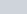[ion:

Reaction type: o

## 82 Laboratory I l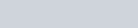Solid copper(ll) hydroxide converts to solid copper(JI) oxide and unknown liquid when heated.

Eqnation:

Reaction type:3. Solid copper(ll) oxide reacts with aqueous sulfuric acid to form aqueous copper(f I)sulfateuation:

Reaction type: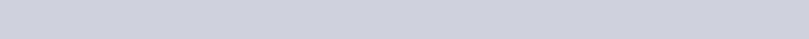Copper(ll) sulfate reacts with zinc metal to form copper metal.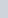Equation: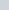Reaction type: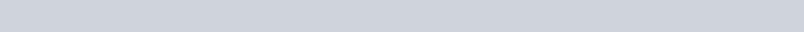1. Crystalline C 6 H 12 O 6 is burned in oxygen, and carbon dioxide and water areformed.Equation:Reaction type:

1. Aqueous magnesium bromide and aqueous sodium hydroxide are mixed, andsolid magnesium hydroxide is formed.

Equation:Reaction type:

Chemical Reactions 83

WRITING COMPLETE MOLECULAR REACTIONS WITH

# SOLUBILITY

Using rhe solubility rules, you may determine if a reaction will OCCUT when two chemicals arc mixed. Use the Solubility Table to determine if each reaction will occur (one of the products must not bc soluble or reaction must form water). Assuming each of the reactants is soluble, write the complete balanced double displacement reaction and indicate if each of the products is solid, liquid, or aqueous. If no reaction will occur because both products are soluble, indicate this fact.

## HCI

1. NaCl + H2S04 —v
2. Na2C03

KCI

1. Li2S04 + caci2—.
2. NaC2HAC)2 +
3. KOH +

# USING SOLUBILITY TO WRITE TOTAL AND NET IONIC

## EQUATIONS

All the previous reactions are in the form of molecular equations. Write reactions 2 and 7 in the form of total ionic and net ionic equations. Remember from your Chapter 7 reading that total ionic shows all ions or molecules (and uses the proper number of each and the correct designations C,aC12 is shown as Ca (aq) + 2 Cl(aq)). Net ionic only shows the ions and molecules that participate in any net reaction.

Reaction 2—total ionic

Reaction 2—net ionic

Reaction 7—total ionic

Reaction 7—net ionic

### 84 Laboratory 1 1Laboratory 1 1 —Chemical Reactions 8)# WORDS TO REACTION FORMULAS

Given the description ofa chemical reaction in words, you can write the correct balanced equation with formulas and, furthermore, figure out what chemical is missing if we give you three out of four molecules. For the following reactions, write the complete balanced reaction, include subscripts; abbreviations for solid, liquid, aqueous, or gas; and state the type of reaction.

l. Aqueous copper(ll) nitrate reacts with aqueous sodium hydroxide to form a precipitatc of copper(ll) hydroxide and an unknown liquid.[ion:

Reaction type: o

## 82 Laboratory I lSolid copper(ll) hydroxide converts to solid copper(JI) oxide and unknown liquid when heated.

Eqnation:

Reaction type:3. Solid copper(ll) oxide reacts with aqueous sulfuric acid to form aqueous copper(f I)sulfateuation:

Reaction type:Copper(ll) sulfate reacts with zinc metal to form copper metal.Equation:Reaction type:1. Crystalline C 6 H 12 O 6 is burned in oxygen, and carbon dioxide and water areformed.Equation:Reaction type:

1. Aqueous magnesium bromide and aqueous sodium hydroxide are mixed, andsolid magnesium hydroxide is formed.

Equation:Reaction type:

Chemical Reactions 83

WRITING COMPLETE MOLECULAR REACTIONS WITH

# SOLUBILITY

Using rhe solubility rules, you may determine if a reaction will OCCUT when two chemicals arc mixed. Use the Solubility Table to determine if each reaction will occur (one of the products must not bc soluble or reaction must form water). Assuming each of the reactants is soluble, write the complete balanced double displacement reaction and indicate if each of the products is solid, liquid, or aqueous. If no reaction will occur because both products are soluble, indicate this fact.

## HCI

1. NaCl + H2S04 —v
2. Na2C03

KCI

1. Li2S04 + caci2—.
2. NaC2HAC)2 +
3. KOH +

# USING SOLUBILITY TO WRITE TOTAL AND NET IONIC

## EQUATIONS

All the previous reactions are in the form of molecular equations. Write reactions 2 and 7 in the form of total ionic and net ionic equations. Remember from your Chapter 7 reading that total ionic shows all ions or molecules (and uses the proper number of each and the correct designations C,aC12 is shown as Ca (aq) + 2 Cl(aq)). Net ionic only shows the ions and molecules that participate in any net reaction.

Reaction 2—total ionic

Reaction 2—net ionic

Reaction 7—total ionic

Reaction 7—net ionic

### 84 Laboratory 1 1